# nikkomycin L(x)

Known as: nikkomycin Lx

National Institutes of Health

## Papers overview

Semantic Scholar uses AI to extract papers important to this topic.
2016
2016
Let \$X\$ be a real or complex Banach space, let \$L(X)\$ be the algebra of all bounded linear operators of \$X\$ into itself and let… Expand
Is this relevant?
2015
2015
• 2015
The problem of finding a fixed point to a nonexpansive operator is an abstraction of many models in numerical linear algebra… Expand
•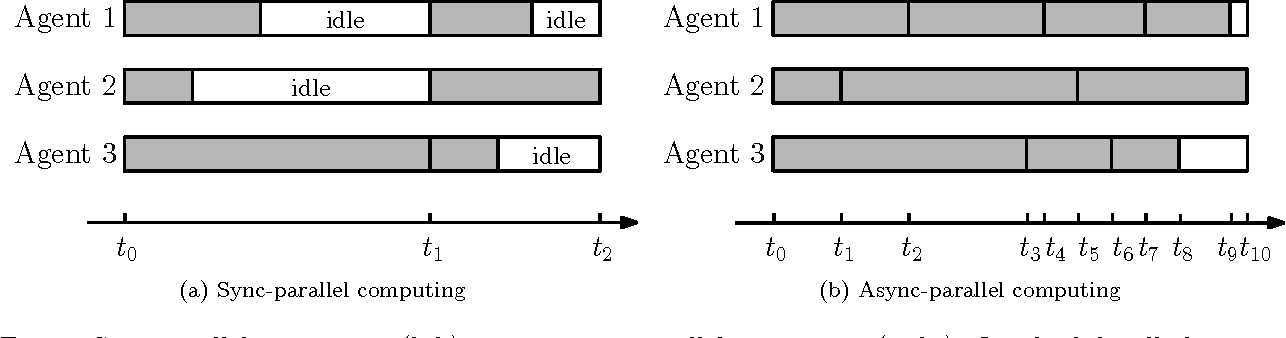•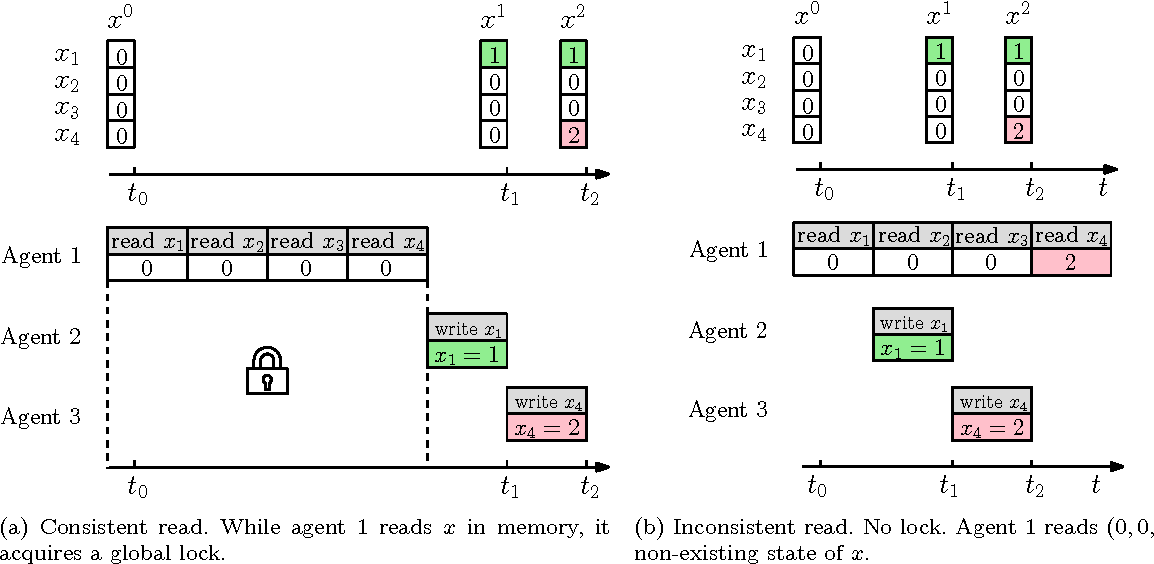•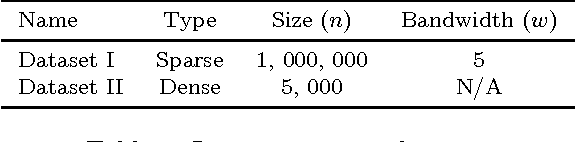•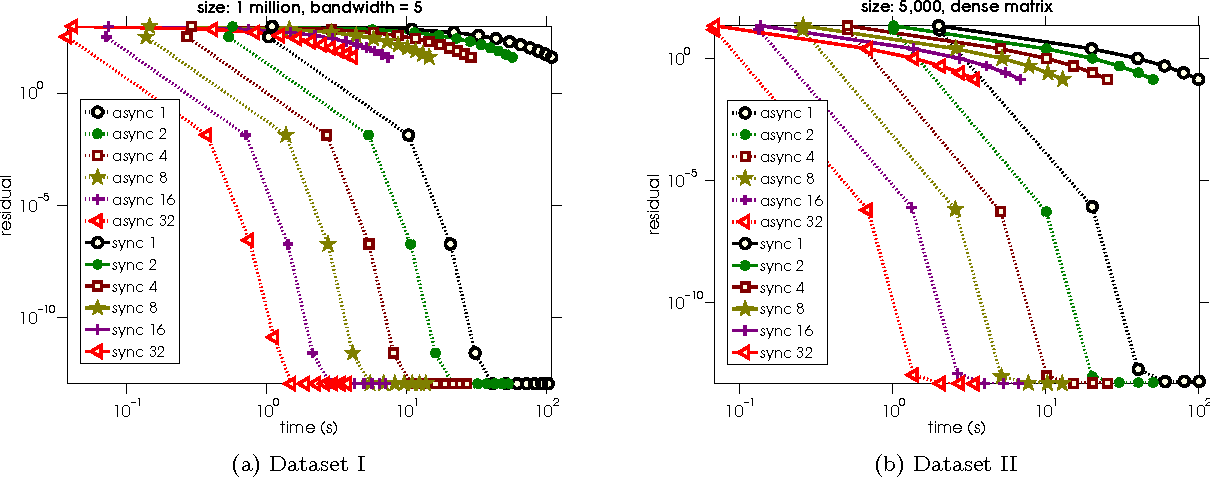•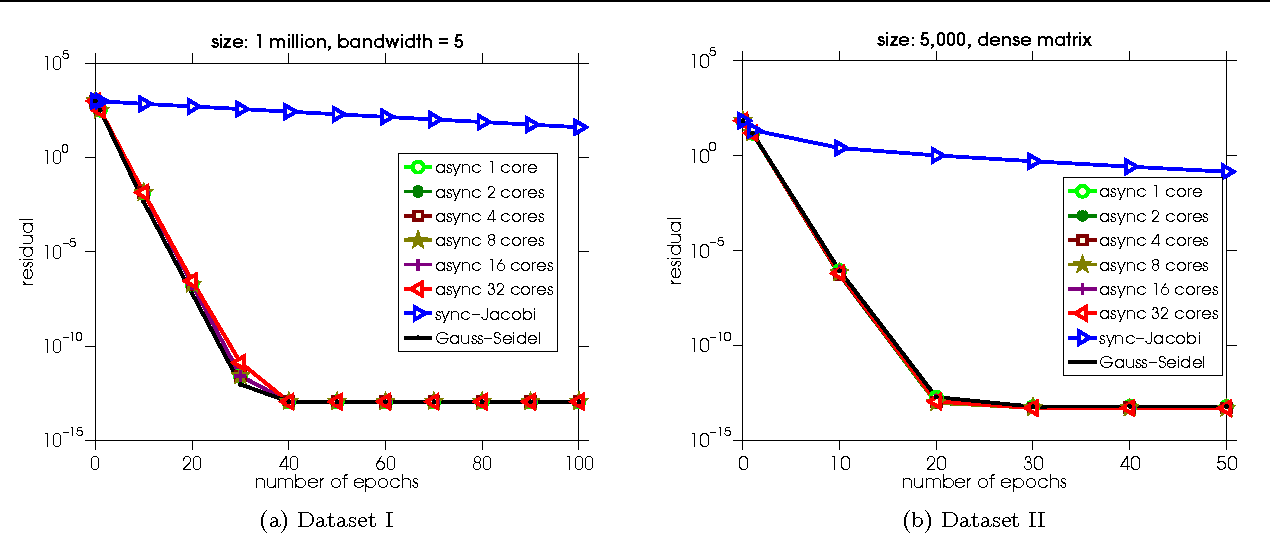Is this relevant?
2014
2014
• Journal of labelled compounds…
• 2014
New 1,4-substituted pyridyl-1,2,3-triazole ligands with pendent phenyl isothiocyanate functional groups linked to the heterocycle… Expand
Is this relevant?
2012
2012
• IEEE Transactions on Systems, Man, and…
• 2012
Traditional learning algorithms use only labeled data for training. However, labeled examples are often difficult or time… Expand
Is this relevant?
2012
2012
In this paper functional equations related to derivations on semiprime rings and standard operator algebras are investigated. We… Expand
Is this relevant?
2010
2010
En este articulo nosotros provamos el seguiente resultado. Sea X un espacio de Banach real o complejo, sea L(X) a algebra de… Expand
Is this relevant?
2005
2005
• 2005
A simplicial complex X is d-Leray if H̃i(Y ; Q) = 0 for all induced subcomplexes Y ⊂ X and i ≥ d. Let L(X) denote the minimal d… Expand
Is this relevant?
2003
2003
This paper develops a general approach to risk management in military applications involving uncertainties in information and… Expand
•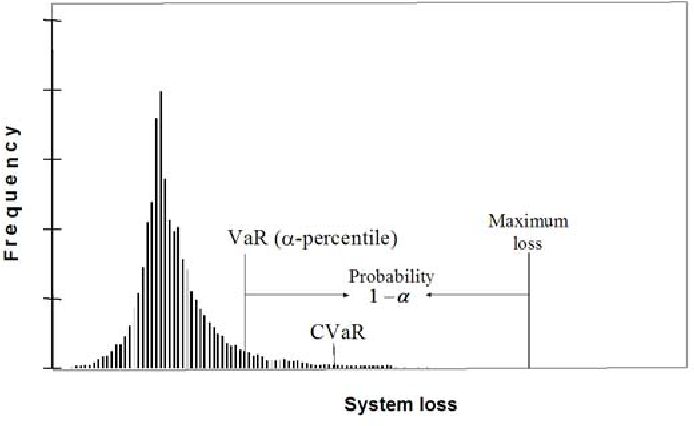•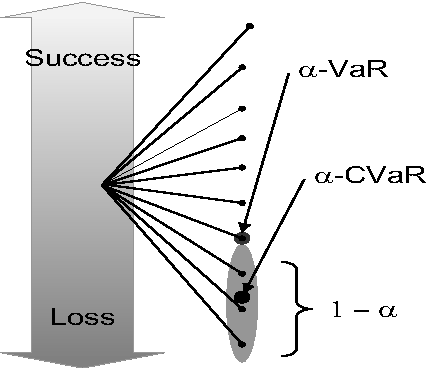•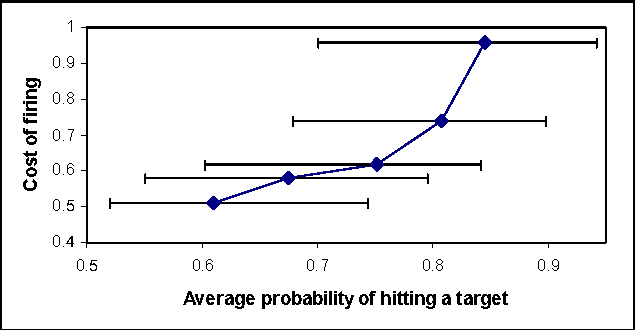•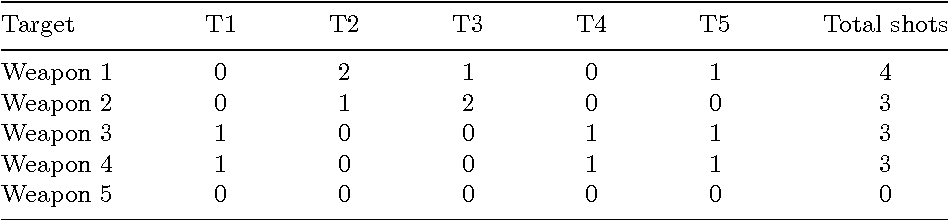•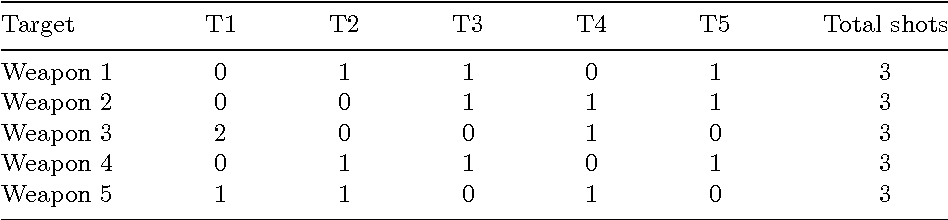Is this relevant?
1995
1995
Norm inequalities for the Riemann-Liouville operator R,f(x) = f( x) 1 A (x-t)f(t)dt and Weyl operator Wrf(x) = f(x oo) Ar I(t-x)f… Expand
Is this relevant?
1990
1990
A semiderivation of a ring R is an additive mapping f:R-+R together with a function g: R —» R such that f(xy) = f(x)g(y) + xf(y… Expand
Is this relevant?